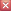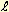Glossary of Terms, Symbols, Equations To return to qm view, close tab or browserabsolute : as indicated by or determined by clocks, measuring rods and observers in an inertial frame at rest in the qm far from any large concentrations of mass/energy, or as determined by observers who allow for the consequences of the qm. (For "absolute velocity" see symbol va below.)

 absolute synchronization of clocks: the synchronization of two clocks so that an observer at rest in the qm could simultaneously observe being midway between the clocks and observe the clocks displaying the same time. (or, when two clocks located next to one another are observed to be synchronized.)

 at-rest : when a body or system is not moving through the qm.

 c : speed of light defined in accordance with orthodox theory. A constant, approximately 300,000,000 m/s in all inertial frames. To see why the notion of c became the foundation on which spacetime theory was built, see * below.

 ca : absolute speed of light through the qm devoid of impeding matter (e.g. air, water, glass) or nearby massive systems (e.g. stars, galaxies) This speed is a constant of nature.

 cag : speed of light through the qm devoid of impeding matter (e.g. air, water, glass) but influenced by nearby massive systems (e.g. stars, galaxies). This influence in a particular location in the qm is determined using the rg equation (see rg symbol below). The speed of light cag at the location is simply rg squared as follows. cag=rg2

 cr : relative speed of light or the speed of a photon or light signal relative to a body or reference frame (which speed must be > 0 ca but < 2 ca).

 crx and crn : maximum and minimum speeds of light respectively relative to a body or reference frame moving through the qm with absolute velocity va. These maximum and minimum speeds of light are given by the following equations where the units of crx, crn, and va are ca. crx=1+va     crn=1−va The ratio crx/crn is the asymmetry ratio N for a body moving with absolute velocity va through the qm. N is the ratio of the number of energy quanta moving in the direction of va to the number of energy quanta moving in the opposite direction. (This is because the quanta of energy moving in the direction of va have a slower speed through the body.) N is also the ratio of the energy of an energy quantum moving in the direction of va to the energy of an energy quantum moving in the opposite direction. (This is because the quanta moving in the direction of va have been blueshifted when emitted, and the quanta moving in the opposite direction have been redshifted.) Further discussion of this is beyond the scope of this website, and it is mentioned here to show these interesting consequences of a body's velocity through the qm. It is also of note that the square root of the product of crx and crn is the physical change ratio rv, that is explained below and specifies important physical characteristics of a body that depend on the body's absolute velocity va.

 E : math symbol meaning 10 raised to whatever power follows E. For example, 3E8 means 3 times 10 raised to the power 8 or 3 times 100,000,000 or 300,000,000. And 3E−8 means 3 times .000,000,01 or .000,000,03.

 inertial reference frame (a.k.a. inertial frame, frame) : an x, y, z coordinate system which has a constant velocity and in which a body at rest remains at rest even when free to move in any direction.

 Ja : absolute joule or 1 Na�ma or 1 kga/9E16.

 kg : virtual kilogram or the apparent mass of a standard 1 kg mass at rest in the inertial reference frame of the observer(c).

 kga : absolute kilogram or a standard 1 kg mass at rest in the qm far from any large concentrations of mass.

 ls : virtual light-second or the distance between two points in an inertial reference frame where the round-trip travel time for a light signal between the two points is two seconds according to clocks in the reference frame.

 LS : absolute light-second or the distance through the qm traveled by light moving with velocity ca for 1 sa. The standard unit of distance in the qm view.

 m : meter or the length of a standard meter rod (which varies depending on its absolute velocity and its orientation relative to the direction of this velocity)

 ma : absolute meter or (1 LS/3E8) or the length of a standard meter rod at rest in the qm far from any large concentration of mass/energy.

 Na : absolute newton or a 1 N force in an inertial frame at rest in the qm.

 observer(c) :  an observer who assumes the speed of light is constant (in vacuum) in all inertial frames.

 observer(cr) :  an observer who assumes the speed of light is not constant in inertial frames moving through the qm.

 photon :  a quantum (i.e. individual unit) of electromagnetic energy having both wave-like and particle-like properties. The energy of a photon is directly proportional to the rate of oscillation of the photon's electromagnetic field. AM radio wave photons have relatively low energy and oscillate at about 100 thousand Hz, where Hz means cycles per second. Visible light photons and X-ray photons oscillate at about a million billion Hz and a billion billion Hz respectively. Gamma-ray photons have very high energy and oscillate at about 100 billion billion Hz!

 qm :  quantum medium. the medium in which photons and other quanta of energy oscillate and through which they are propagated. Possibly the same aspect of nature as the quantum vacuum of quantum mechanics theory. (Similar to the "electromagnetic ether" envisioned by Maxwell and Dirac and in which photons, electrons, and other "particles" were oscillations. But the term "electromagnetic" suggests the exclusion of some kinds of energy in the medium, so we no longer use this term when referring to the medium and the energy therein.)

 radar ranging : the determination of the distance to a target (e.g. airplane, spaceship, moon, planet) by transmitting electromagnetic signals (photons) to the target, receiving the reflected signal from the target, and determining the signal travel time and distance to the target.

 rg :  gravity-causing, photon-slowing physical change ratio for a system due to its proximity to a large concentration of mass/energy m (e.g. star, Earth). It is the ratio of the rate of round-trip energy exchange in the system to the rate in the absence of m. It is the ratio of the rate of evolution of a clock or other process in the system to the rate of evolution in the absence of m. It is also the ratio of the length and width and height of the system to the length, width, and height in the absence of m. Also, it is the ratio of the system's mass to its mass when not affected by m. Ratio rg is determined by the following equation where m is the mass of the concentration of mass/energy in kga,is the distance from m in absolute light second units LS, and G is the Newtonian gravitational constant (which is 6.67E-11 / 27E24 = 2.47E-36 when distances are in LS units).rv :  physical change ratio for a system due to its velocity through the qm. It is the ratio of rate of round-trip energy exchange in the system to the at-rest rate. It is the ratio of the rate of evolution of a clock or other process in the system to the rate of evolution when at rest in the qm. It is also the ratio of the length of the system in the direction of va to the length when the system is at rest in the qm. Also, it is the ratio of the system's at-rest mass to its mass when moving through the qm with absolute velocity va. Ratio rv, which is equal to the square root of the product of crx and crn, can be determined using the following equation where va is the absolute velocity of the system or body.s : virtual second or one second on an atomic clock or other precise clock moving through the qm (or slowed by any large concentration of mass).

 sa : absolute second or one second on an atomic clock at rest in the qm far from any large concentration of mass.

 spacetime theory : Relativity theory and its consequences including conclusions that distance, time, and mass are relative because there can be no absolute units of distance, time, and mass, and that gravity is the result of curvature of spacetime caused by mass/energy.

 ta : absolute time. the time in sa before or after a specified event that occurs at ta=0 sa, or the time in sa between two events.

 v : virtual velocity or an observed velocity in a reference frame when using the virtual units of distance (ls or m) and time (s) in the reference frame. The symbol vCB means the observed virtual velocity of a body or reference frame C relative to a body or frame B.

 va : absolute velocity. the velocity of a body or reference frame through the qm. This velocity can be expressed in terms of ca (e.g. va = .5 ca means half the speed of light through the qm). Note: Absolute velocity units (e.g. ca) are also used to specify absolute relative velocities. The symbol vCBa means the absolute velocity of a body or reference frame C relative to a body or frame B. Absolute relative velocities are determined using absolute units of distance (LS or ma) and time (sa).

 virtual synchronization of clocks: the synchronization of two clocks in an inertial frame moving through the qm so that an observer(c) in the frame could simultaneously observe the clocks being the same distance from the observer and observe the clocks displaying the same time. (or, when two clocks in an inertial frame moving through the qm are separated after having been observed to be both next to one another and synchronized.)

 * The following is from A. Einstein's Relativity: The Special and the General Theory, pages 17-18.

 VII THE APPARENT INCOMPATIBILITY OF THE LAW OF PROPAGATION OF LIGHT WITH THE PRINCIPLE OF RELATIVITY      There is hardly a simpler law in physics than that according to which light is propagated in empty space. Every child at school knows, or believes he knows, that this propagation takes place in straight lines with a velocity c=300,000 km./sec. At all events we know with great exactness that this velocity is the same for all colours, because if this were not the case, the minimum of emission would not be observed simultaneously for different colours during the eclipse of a fixed star by its dark neighbour. By means of similar considerations based on observations of double stars, the Dutch astronomer De Sitter was also able to show that the velocity of propagation of light cannot depend on the velocity of motion of the body emitting the light. The assumption that this velocity of propagation is dependent on the direction "in space" is in itself improbable.      In short, let us assume that the simple law of the constancy of the velocity of light c (in vacuum) is justifiably believed by the child at school. Who would imagine that this simple law has plunged the conscientiously thoughtful physicist into the greatest intellectual difficulties? Let us consider how these difficulties arise.It is true that the assumed constant speed of light c leads to great difficulties and that spacetime theory is a remarkable way of resolving some of them. But spacetime theory itself presents difficulties, as physics students learn. It is a complex theory that requires denying the existence of distances, times, and masses that are independent of who is observing them, and it causes other difficulties and confusion. It leads to unrealistic ideas, like going back in time and changing the course of history. Are not the difficulties caused by assuming constant light speed c reason to question the assumption?

 To return to qm view, close tab or browser